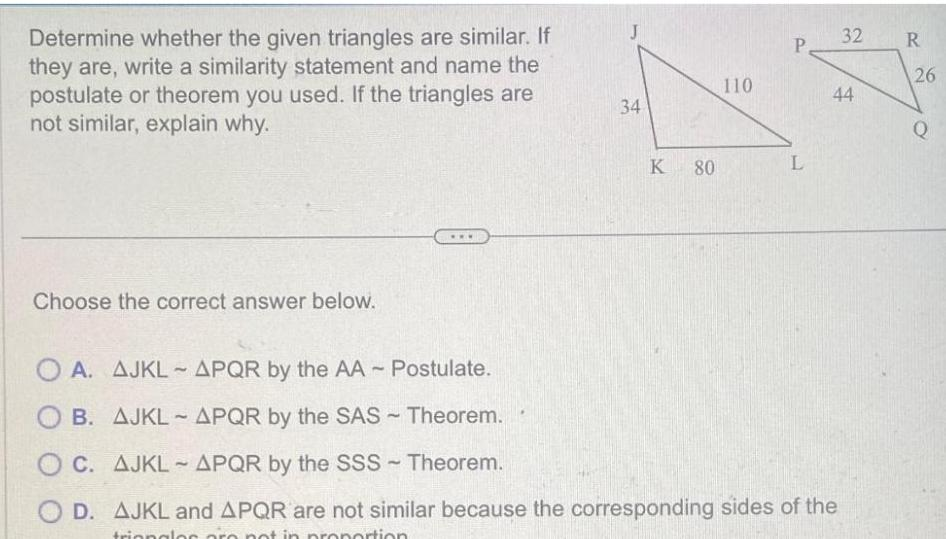Question:

# Determine whether the given triangles are similar If they

Last updated: 11/21/2023Determine whether the given triangles are similar If they are write a similarity statement and name the postulate or theorem you used If the triangles are not similar explain why Choose the correct answer below 34 K 80 110 P L OA AJKL APQR by the AA Postulate OB AJKL APQR by the SAS Theorem OC AJKL APQR by the SSS Theorem OD AJKL and APQR are not similar because the corresponding sides of the triangles are not in proportion 32 R 44 26 Q